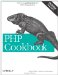# Recipe 4.7. Appending One Array to Another

#### 4.7.1. Problem

You want to combine two arrays into one.

#### 4.7.2. Solution

Use array_merge( ):

`\$garden = array_merge(\$fruits, \$vegetables);`

#### 4.7.3. Discussion

The array_merge( ) function works with both predefined arrays and arrays defined in place using array( ) :

`\$p_languages = array('Perl', 'PHP'); \$p_languages = array_merge(\$p_languages, array('Python')); print_r(\$p_languages); Array (      => PHP      => Perl      => Python )`

Accordingly, merged arrays can be either preexisting arrays, as with \$p_languages, or anonymous arrays, as with array('Python').

You can't use array_push( ), because PHP won't automatically flatten out the array into a series of independent variables, and you'll end up with a nested array. Thus:

`array_push(\$p_languages, array('Python')); print_r(\$p_languages); Array (      => PHP      => Perl      => Array         (              => Python         ) )`

Merging arrays with only numerical keys causes the arrays to get renumbered, so values aren't lost. Merging arrays with string keys causes the second array to overwrite the value of any duplicated keys. Arrays with both types of keys exhibit both types of behavior. For example:

`\$lc = array('a', 'b' => 'b'); // lower-case letters as values \$uc = array('A', 'b' => 'B'); // upper-case letters as values \$ac = array_merge(\$lc, \$uc);  // all-cases? print_r(\$ac); Array (      => a     [b] => B      => A )`

The uppercase A has been renumbered from index 0 to index 1, to avoid a collision, and merged onto the end. The uppercase B has overwritten the lowercase b and replaced it in the original place within the array.

The + operator can also merge arrays. The array on the right overwrites any identically named keys found on the left. It doesn't do any reordering to prevent collisions. Using the previous example:

`print_r(\$uc + \$lc); print_r(\$lc + \$uc); Array (      => a     [b] => b ) Array (      => A     [b] => B )`

Since a and A both have a key of 0, and b and B both have a key of b, you end up with a total of only two elements in the merged arrays.

In the first case, \$a + \$b becomes just \$b, and in the other, \$b + \$a becomes \$a.

However, if you had two distinctly keyed arrays, this wouldn't be a problem, and the new array would be the union of the two arrays.

Documentation on array_merge( ) at http://www.php.net/array-merge .PHP Cookbook: Solutions and Examples for PHP Programmers
ISBN: 0596101015
EAN: 2147483647
Year: 2006
Pages: 445

Similar book on Amazon